# Examples of how to set road levels at interchanges

Example 1

Illustrates how road sections are divided up and how levels A and B are set at a multi-level interchange (see Section 3.3.2.5. Level (A) and Level (B)) on the example of a cloverleaf interchange (at the intersection of the M-4 and R-140 highways).

Example 2

Illustrates how road sections are divided up and how levels A and B are set at a multi-level interchange (in the Antwerp metropolitan area).

## Example 1

Illustration of how road sections are divided up and how levels A and B are set (see Section 3.3.2.5. Level (A) and Level (B)) at a cloverleaf interchange (at the intersection of the M-4 and R-140 highways); the figures show the successive stages of how levels A and B are set along highways and at interchange ramps.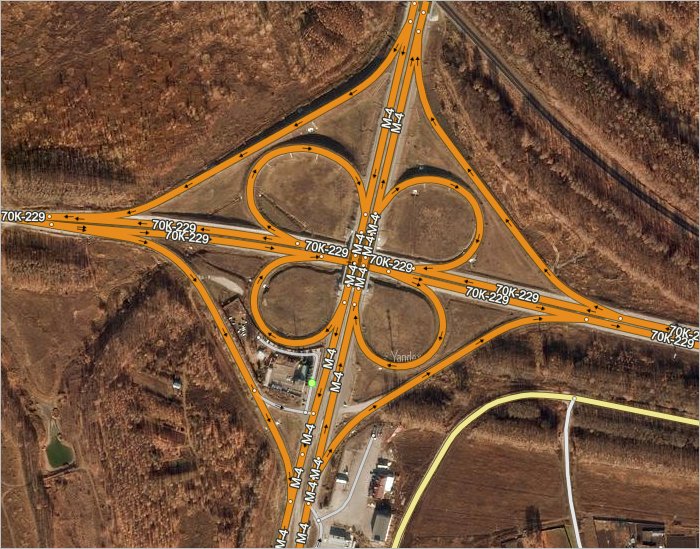Figure 1. Photo of the interchange with a map of the road network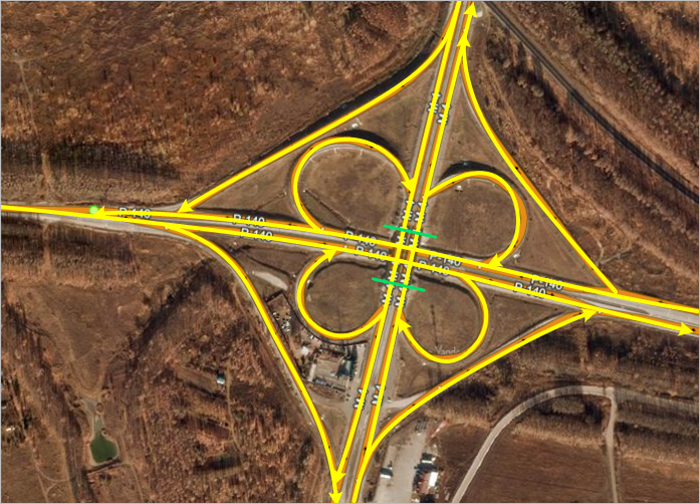Figure 2. Road network map. The arrows show the traffic direction and the drawing direction (A → B) ; the green lines indicate the bridge abutment where the M-4 highway intersects with the P-140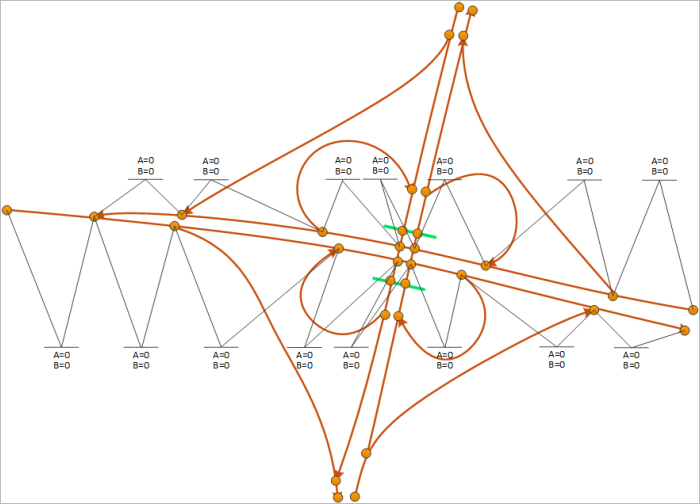Figure 3. Mapping levels A and B of P-140 highway sections (the brown circles represent road intersections); all levels equal zero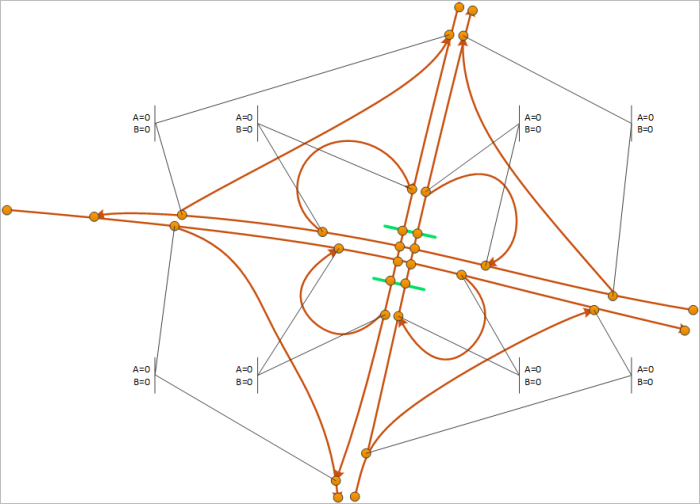Figure 4. Mapping levels A and B of P-140 and M-4 exit ramps (the brown circles represent road intersections); all levels equal zero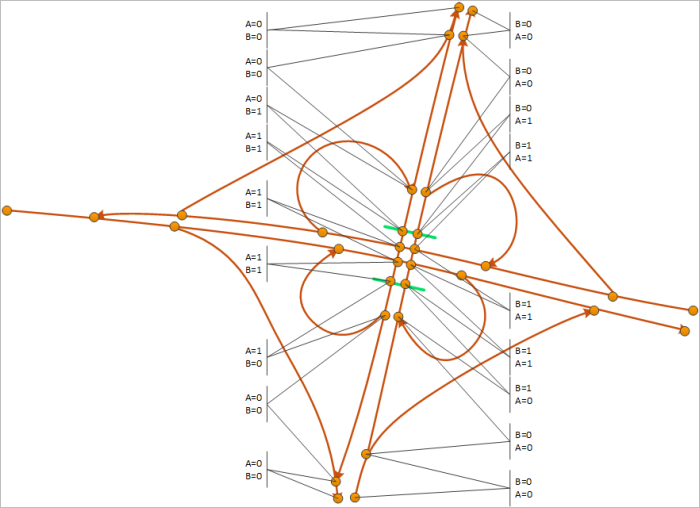Figure 5. Mapping levels A and B of M-4 highway sections (the brown circles represent road intersections). Note that now the values of levels A and B have gone from zero to one as the highway passes over the bridge.

## Example 2

Illustration of how road sections are divided up and how levels A and B are set (see Section3.3.2.5. Level (A) and Level (B)) at a multi-level interchange (in the Antwerp metropolitan area ). The figures show the successive stages of mapping levels A and B.